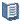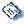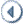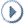Oracle® Fusion Applications Project Management Implementation Guide 11g Release 5 (11.1.5) Part Number E20384-05ContentsContactUsPreviousNext

# 23 Project Foundation Configuration: Define Rate Schedules and Costing Rules

This chapter contains the following:

Rate Schedule Types: Explained

Labor Cost Rates: How They Are Determined

Labor Costing Rules: Explained

Defining Labor Costing Rules: Example

Creating Labor Costing Multipliers: Examples

Setting Up Labor Costing Overrides: Critical Choices

Organization Costing Rule Components: How They Work Together

Extensions for Define Rate Schedules and Costing Rules

## Rate Schedule Types: Explained

Schedule types determine usage for rates within rate schedules. You specify a schedule type for rate schedules created for costing, billing, or planning purposes in Oracle Fusion Projects.

The schedule types are:

• Job

• Person

• Nonlabor

• Resource class

### Job

Job rate schedules contain rates used to calculate amounts for the following types of labor transactions:

• Costing

• Billing (invoice and revenue)

• Planning

• Budgeting

• Forecasting

• Transfer price

The rate is based on the standard hourly rate assigned to a job title in Oracle Fusion Human Capital Management.

If you are using planning rates for financial or project planning, you can select a specific job rate schedule when configuring rate settings at the plan type or project level. Job rate schedules are used if rates cannot be derived from the person labor rate schedule.

When creating a job schedule type, you must select a job set from Oracle Fusion Human Capital Management. The job set is the source of jobs in your rate schedule. Assign rates or markup percentages to jobs in the rate schedule.

### Person

Person schedules contain raw cost rates and billing rates or markup percentages for labor transactions and transfer price amounts. The rate that calculates the cost or billing amount for a project transaction is based on the standard hourly rate or markup percentage assigned to a person, or the job or organization assigned to the person in the schedule. The job or organization is based on the person's assignment in Oracle Fusion Human Capital Management.

You have the option of assigning rates to the following:

• Person

• Person and job

• Person, job and organization

If you assign a rate to a person and job combination, that rate has precedence over the person rate. If you assign a rate to a person, job and organization combination, that rate has precedence over the person rate or person and job combination.

If you are using planning rates for financial or project planning, you can select a specific person rate schedule when configuring rate settings at the plan type or project level. Person rate schedules are used if rates cannot be derived from the labor rate schedule.

### Nonlabor

Nonlabor rate schedules contain rates or markup percentages that calculate cost, bill, revenue, plan, budget, forecast, or transfer price amounts for nonlabor resources.

Enter a rate or markup percentage for expenditure types with the Rate Required option enabled. Otherwise, assign it only a markup percentage. Assign rates to nonlabor resources and optionally define rates for nonlabor resource organizations.

If you are using planning rates for financial or project planning, you can select a specific nonlabor rate schedule when configuring rate settings at the plan type or project level.

### Resource Class

Resource class schedules contain the planning rates or markup percentages for a resource class or a combination of resource class and organization. You optionally assign a resource class schedule to a project plan or financial plan (budgets and forecasts) at the plan type level or version level. The resource class rate schedule determines rates for the associated resources if the rates cannot be derived elsewhere.

Enter a rate or markup percentage for each resource class in the rate schedule. Optionally, assign the rate or markup percentage to a specific organization for a resource class.

## Labor Cost Rates: How They Are Determined

Oracle Fusion Projects uses cost rates to calculate the raw cost for expenditure items. The application determines a cost rate for each expenditure item and calculates the raw cost during cost distribution processing, unless you import the raw cost for expenditure items.

## How Labor Cost Rates Are Determined

When you assign a labor costing rule and a rate schedule to an organization, Oracle Fusion Projects applies the following rules in the order presented to determine the labor costing rule for each transaction.

1. Oracle Fusion Projects determines whether any labor costing overrides exist for the employee who is associated with the expenditure item. The application uses the effective dates for the labor costing overrides to determine whether an override is active on the expenditure item date. A labor costing override can have either an overriding cost rate or an overriding rate schedule. If a labor costing override applies, then Oracle Fusion Projects uses it to determine the cost rate.

Labor expenditure items always have a unit of measure of Hours.

For labor transactions, the application searches for a labor costing override in the following order.

1. Person, job, and organization combination

2. Person and job combination

3. Person

2. If no override exists, Oracle Fusion Projects uses an organization costing rule to determine the cost rate. The following logic determines the cost rate. At each level, the application searches for a rule with a date range that includes the expenditure item date.

1. Organization costing rule for the expenditure organization

The application searches for an active costing rule assigned to the organization of the expenditure item on the date of the transaction.

2. Organization costing rule for the parent expenditure organization

If an organization costing rule for the expenditure organization is not found, the application searches for a rule assigned to the parent organization of the expenditure organization, and continues up the project expenditure organization hierarchy until a rule is found.

You specify the project expenditure organization hierarchy for the business unit during implementation. If an organization has multiple parent organizations, and a rule is assigned to more than one parent, the application uses the rule assigned to the lowest level parent organization.

3. Organization costing rule for the business unit

If no costing rule is found for the expenditure organization and parent organization, the application uses the costing rule assigned to the business unit for the expenditure.

Oracle Fusion Projects applies the costing rule to determine the cost rate for the expenditure item. You can associate either a rate schedule, or a Labor Costing extension with an organization labor costing rule, to determine the cost rate.

## Labor Costing Rules: Explained

A labor costing rule determines how an employee is paid. For example, you can define a labor costing rule for pay types such as exempt, nonexempt, uncompensated, compensated, and hourly.

When an employee charges time to a project, Oracle Fusion Projects processes the labor hours according to the employee's labor costing rule. For example, if an employee's labor costing rule is Hourly, the employee is eligible for overtime pay; if the employee's labor costing rule is Exempt, the employee is not eligible for overtime pay.

Following are the key components of a labor costing rule:

• Costing method

• Overtime labor costing multipliers

• Default overtime transaction attributes

### Costing Method

For labor costing rules with the Rates costing method, labor costs are calculated for entered hours using hourly cost rates.

For labor costing rules with the Extension costing method, labor costs are calculated by the Labor Costing extension. When you use the Extension costing method, you are not required to maintain hourly cost rates in Oracle Fusion Projects.

### Overtime Labor Costing Multipliers

If your employees enter overtime hours manually, you can assign cost multipliers to overtime expenditure types. When you use the Rates costing method, and a transaction is charged to an expenditure type that has an assigned multiplier, the application applies the multiplier as labor costs are calculated.

To calculate rates for overtime expenditure items, before you define labor costing rules, you must define an expenditure type with the Overtime expenditure type class.

If overtime hours are calculated using the Overtime Calculation extension, you can specify the default expenditure types for the overtime expenditure items generated by the extension.

### Default Overtime Transaction Attributes

If overtime hours are created by the Overtime Calculation extension, you can specify the default business unit, project, and task that incur the overtime costs generated by the extension. These attributes apply only if you use the Overtime Calculation extension to calculate overtime hours.

## Defining Labor Costing Rules: Example

This example illustrates setting up labor costing rules to calculate overtime labor costs.

### Scenario

You are asked to set up labor costing rules to calculate overtime labor costs for nonexempt and hourly employees for the InFusion Corporation.

#### Overtime Costs

InFusion Corporation uses the Overtime Calculation extension to calculate overtime hours for nonexempt employees. All overtime premium costs for nonexempt employees are charged to an indirect project.

Hourly employees are required to enter overtime hours manually. All labor costs, including overtime premiums, are charged to the project and task indicated on the timecard.

#### Analysis

For nonexempt employees, the expenditure types for overtime transactions created using the Overtime Calculation extension are derived from the overtime labor cost multipliers that are assigned to the labor costing rule.

For hourly employees, when time is charged to an overtime expenditure type, the application applies the costing multiplier assigned to the labor costing rule when labor costs are calculated.

#### Labor Costing Rule Details

The following table shows the labor costing rules for InFusion Corporation:

Labor Costing Rule

Costing Method

Project

Nonexempt

Rates

Overtime

Time and a Half

Hourly

Rates

The following table shows the overtime labor cost multipliers that are associated with the labor costing rules:

Labor Costing Rule

Expenditure Type

Labor Cost Multiplier

Nonexempt

Overtime

Time and a Half

Hourly

Overtime

Time and a Half

Hourly

Double Time

## Creating Labor Costing Multipliers: Examples

A labor costing multiplier is a value by which Oracle Fusion Projects multiplies an employee's labor cost rate to calculate the employee's overtime premium cost rate. This is represented by the formula: labor cost rate * labor costing multiplier = overtime premium labor cost rate.

Oracle Fusion Projects then multiplies the overtime premium labor cost rate by the number of overtime hours that an employee works to calculate the overtime premium for the employee. This is represented by the formula: overtime premium labor cost rate * overtime hours = overtime premium.

### Labor Costing Multipliers

You define a labor costing multiplier for each kind of overtime your business uses such as double time, or time and a half. For example, if you pay an employee double time for all overtime hours, you define a labor cost multiplier of 1.0. You multiply the employee's labor cost rate by 1.0 to calculate the employee's overtime premium labor cost rate. If you pay an employee time and a half for all overtime hours, you define a labor cost multiplier of 0.5 to calculate the employee's overtime premium labor cost rate. An employee's total labor cost is the overtime premium plus the total number of hours that employee worked multiplied by the employee's labor cost rate. This is represented by the formula: overtime premium + (total hours x labor cost rate) = total labor cost.

The following table shows examples of labor cost multipliers for double time, time and a half, and uncompensated overtime. The negative multiplier for uncompensated overtime reverses the cost of any overtime hours for the individuals who do not get paid overtime.

Labor Costing Multiplier Name

Multiplier

Double Time

1.0

Time and a Half

0.5

Uncompensated Overtime

-1.0

## Setting Up Labor Costing Overrides: Critical Choices

You can override the labor costing definition for individual employees and contingent workers for a business unit. The application uses a labor costing override to determine the labor rate for cost transactions, and to plan, budget, and forecast transactions when you use an actual plan type.

Following are the key implementation concepts for setting up labor costing overrides:

• Override for a Person, Job, And Organization

• Override by Rate, Rate Schedule, or Extension

• People with Future-Dated and Terminated Assignments

### Override for a Person, Job, and Organization

You specify the labor costing override for a person, or for a more granular override, specify a combination of person and job, or person, job, and organization.

The job and organization represent the human resources job and organization of the person. The jobs that you can select to associate with a labor costing override are based on the person value. The organizations that you can select are based on the job value. When you select a job, an organization is automatically selected by default. The default organization value is based on the selected job assignment. You can accept the default organization for the labor costing override, select a different organization that is associated with the job and person, or remove the organization so the labor costing override is for person and job combination.

In the example shown in the following table, a person is assigned the Nurse job in two different organizations. When you select Nurse to create a labor costing override, the application automatically selects the default organization General Hospital. You can accept the General Hospital default organization, or change the selection to the University Hospital organization, to create an override for the person, job, and organization combination. Alternatively, you can remove the organization value to create an override for the person, job, and any organization.

Job

Department

Organization

Person Type

Primary

Nurse

General Hospital

Employee

Yes

Nurse

Optometry

University Hospital

Contingent Worker

No

### Override by Rate, Rate Schedule, or Extension

When you use a labor costing override, an override rate, rate schedule, or extension takes precedence over a standard rate schedule for the business unit.

When you select a labor costing rule for the override, if the costing method of the rule is Extension, then the override type is Extension by default. If you do not select a labor costing rule, you cannot select an override type of Extension.

### People with Future-Dated and Terminated Assignments

You can set up labor costing overrides for people with a start date in the future if you enable the People with Future Effective Start Dates as Project Members Allowed profile option. You can set up labor costing overrides for people with terminated assignments if you enable the Number of Days to Display People with Terminated Assignments profile option.

## Organization Costing Rule Components: How They Work Together

Use organization costing rules to assign labor costing rules, labor cost rate schedules, and nonlabor cost rate schedules, to business units or specific expenditure organizations. The schedule type on the organization costing rule determines if a labor cost rate schedule or nonlabor cost rate schedule is assigned.

The following components work together to determine organization costing rules.

• Business unit and expenditure organization

• Schedule type

• Labor costing rule and cost rate schedule

• Default overtime transaction attributes

### Business Unit and Expenditure Organization

If you select a business unit for an organization costing rule, Oracle Fusion Projects limits the expenditure organizations that you can select to just the organizations that belong to the project expenditure organization hierarchy for the selected business unit. If you do not select a business unit for an organization costing rule, you can select any expenditure organization that belongs to any project expenditure organization hierarchy.

If you assign an organization labor costing rule to an organization that is not classified as a project expenditure organization, the labor costing rule applies to all organizations that are below the specified organization in the project expenditure organization hierarchy, unless you assign a labor costing rule to an organization at a lower level in the hierarchy.

For example, assume a hierarchy has three organizations: Organization 1, Organization 2, and Organization 3. Organization 1 is the parent of Organization 2. Organization 2 is the parent of Organization 3. Organization 3 is the only organization that is classified as a project expenditure organization. If you assign organization labor costing rules only to Organization 1 and Organization 2, the rule that you assign to Organization 2 takes precedence for Organization 3.

### Schedule Type

Use the Labor schedule type to use labor cost rate schedules to calculate costs for labor transactions such as timecards. If you select a schedule type of Labor, you must enter a labor costing rule.

Use the Nonlabor schedule type to use nonlabor cost rate schedules to calculate costs for nonlabor transactions such as miscellaneous or usage transactions. If you select a schedule type of Nonlabor, you must enter a nonlabor cost rate schedule.

If the plan type is configured to use actual rates, the pricing engine also uses these rates for planning, budgeting, and forecasting transactions.

### Labor Costing Rule and Cost Rate Schedule

To calculate labor costs, you must assign a labor costing rule to each expenditure organization. You assign a labor costing rule to the organization costing rule. If the labor costing rule has a costing method of Rates, you must also assign a cost rate schedule that defines the hourly cost rates for employees in the selected organization.

The labor costing rules and cost rate schedules that you assign to an organization apply to all employees in the organization.

### Default Overtime Transaction Attributes

If an organization uses the Overtime Calculation extension, you can enter default overtime projects and tasks for the system-generated transactions.

## Extensions for Define Rate Schedules and Costing Rules

### Labor Costing Extension

Use Labor Costing Extensions to derive raw cost amounts for individual labor transactions. Examples of labor costing extensions that you may define are:

• Standard cost rate by job

• Capped labor cost rates

• Multiple cost rates per employee

The extension is identified by the following items:

Extension Component

Name

Body template

pjf_client_extn_costing.pkb

Specification template

pjf_client_extn_costing.pkh

Package

PJF_CLIENT_EXTN_COSTING

Procedure

calc_raw_cost

Important

Do not change the name of the extension procedures or parameters. Also, do not change the parameter types or parameter order in your procedure. After you write a procedure, compile it and store it in the database.

Parameters

In the following table is information about parameters for this extension:

Parameter Name

Description

Transaction type

The default value is ACTUAL. Other values are BUDGET and FORECAST.

Transaction interface identifier

Passed in when the transaction type is ACTUAL.

Expenditure type class

Resource assignment identifier

Passed in when the transaction type is BUDGET or FORECAST and when invoked for labor planning transactions when actual rates are used.

Transaction raw cost

The raw cost amount that your procedure calculates is assigned to the x_raw_cost parameter. Leave this value blank to use the standard costing method which uses the employee's hourly cost rate. If you pass a value to this parameter, Oracle Fusion Projects calculates the raw cost rate of the transaction using the x_raw_cost parameter value divided by the number of hours.

Transaction currency

Currency in which the transaction is incurred.

Status

Use the x_status parameter to handle error conditions for your procedure. This parameter indicates the processing status of your extension as shown:

• x_status is 0: The extension executed successfully.

• x_status is less than 0: An application error occurred and the process did not complete.

• x_status is greater than 0: An application error occurred and the process did not complete.

Error details

An application error occurs if the extension fails to return a value for the raw cost rate or amount. Oracle Fusion Projects writes an error message to the process log file.

Cost rate

Parameter returned by the extension.

You can use Labor Costing Extensions to implement unique costing methods other than the standard method, which calculates raw cost using the number of hours multiplied by the employee's hourly cost rate. For example, you may want to calculate the raw cost using a capped labor rate for specific employees.

Oracle Fusion Projects processes Labor Costing Extensions before calculating standard raw cost amounts. If Oracle Fusion Projects encounters a Labor Costing Extension that derives the raw cost amount of a labor transaction, it skips the standard raw cost calculation section for that transaction.

### Overtime Calculation Extension

Use the Overtime Calculation Extension to define your own rules to implement company-specific overtime calculation policies.

The extension is identified by the following items:

Extension Component

Name

Body template

pjc_client_extn_calc_overtime.pkb

Specification template

pjc_client_extn_calc_overtime.pkh

Package

pjc_client_extn_calc_overtime

Oracle Fusion Projects provides sample business rules in the Overtime Calculation Extension. You can use the sample to understand the extension, and then make appropriate changes to meet your business needs.

The sample business rules in the extension calculate overtime costs and charge them to an indirect project other than the project where the labor is charged.

Note

If you want to charge overtime to the project where the labor is charged, consider creating items using the Related Transaction Extension.

Points to consider when implementing the Overtime Calculation Extension:

• Define all overtime expenditure types with an end date.

• Base automatic overtime calculation on weekly overtime rules. Oracle Fusion Projects is designed to process weekly time cards. All expenditure item dates on a time card must be within the expenditure week ending date of the time card. Therefore, automatic overtime calculation is most easily performed based on weekly overtime rules.

The sample business rules in the Overtime Calculation Extension follows these steps to process overtime:

• Determines all employees and corresponding weeks which may include new overtime to process. The Overtime Calculation Extension calculates and creates overtime only for employees with time cards processed in the Import and Process Cost Transactions process that calls the Overtime Calculation Extension. These employees and weeks are identified by the request ID of the straight time expenditure items that are costed before the Overtime Calculation Extension is called.

• Sums the hours required to calculate overtime for identified employees and weeks. The standard Overtime Calculation Extension sums the total hours for the week and the total hours for each day of the week, relying on the time card entry validation rule that all labor expenditure item dates must be within the expenditure week ending date of the time card.

• Calculates overtime hours based on the hours worked, the employee's labor costing rule, and other criteria you specify. The standard Overtime Calculation Extension calculates overtime for an employee and a week based on the employee's labor costing rule.

• Creates overtime expenditure items for each type of overtime for which the employee is eligible. The overtime item is charged to the overtime project and appropriate overtime task that is specified in the Overtime Calculation Extension using the overtime expenditure type defined for the employee's labor costing rule. The expenditure item date is set to the week ending date.

The extension uses the same batch name as the source straight time expenditure items to create the new overtime expenditure items.

• Lists overtime transactions created by this extension in the Import and Process Cost Transactions Execution Report.

The new overtime items are costed after the Overtime Calculation Extension completes, within the Costing stage in the Import and Process Cost Transactions process.